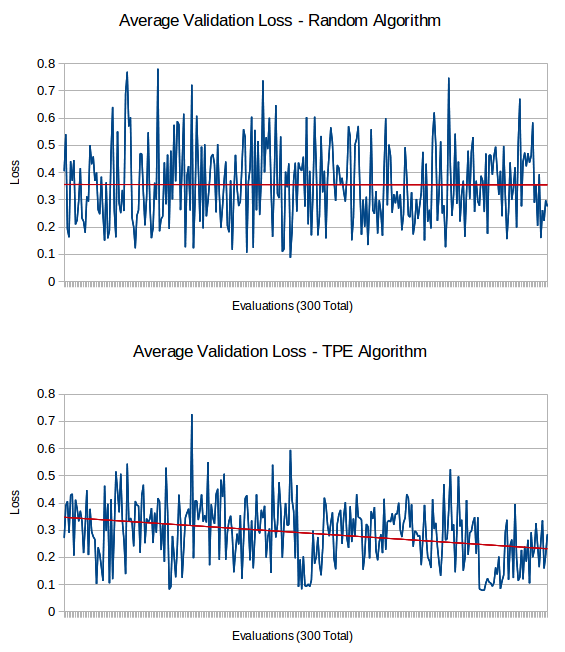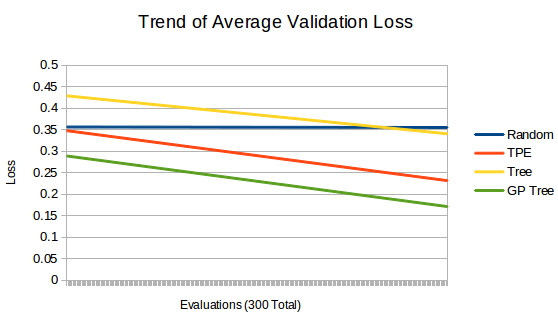# hyperopt-sklearn

Hyper-parameter optimization for scikit-learn

## What is Hyperopt-sklearn?

Finding the right classifier to use for your data can be hard. Once you have chosen a classifier, tuning all of the parameters to get the best results is tedious and time consuming. Even after all of your hard work, you may have chosen the wrong classifier to begin with. Hyperopt-sklearn provides a solution to this problem.

## Usage

```from hpsklearn import HyperoptEstimator

# ...

# Create the estimator object
estim = HyperoptEstimator()

# Search the space of classifiers and preprocessing steps and their
# respective hyperparameters in sklearn to fit a model to the data
estim.fit( train_data, train_label )

# Make a prediction using the optimized model
prediction = estim.predict( unknown_data )

# Report the accuracy of the classifier on a given set of data
score = estim.score( test_data, test_label )

# Return instances of the classifier and preprocessing steps
model = estim.best_model()
```

## Search Algorithms

Any search algorithm available in hyperopt can be used to drive the estimator. It is also possible to supply your own or use a mix of algorithms. The number of points to evaluate before returning, as well as an optional timeout (in seconds) can be used with any search algorithm.

```from hpsklearn import HyperoptEstimator
from hyperopt import tpe

estim = HyperoptEstimator( algo=tpe.suggest,
max_evals=150,
trial_timeout=60 )
```

Search algorithms available so far:

• Random Search
• Tree of Parzen Estimators (TPE)
• Annealing
• Tree
• Gaussian Process Tree

## Classifiers

If you know what type of classifier you wish to use on your dataset, you can let hpsklearn know and it will only search in the parameter space of the given classifier.

```from hpsklearn import HyperoptEstimator, svc

estim = HyperoptEstimator( classifier=svc('mySVC') )
```

You can also provide sets of classifiers, and optionally choose the probability of the estimator picking each one.

```from hpsklearn import HyperoptEstimator, random_forest, svc, knn
from hyperopt import hp

clf = hp.pchoice( 'my_name',
[ ( 0.4, random_forest('my_name.random_forest') ),
( 0.3, svc('my_name.svc') ),
( 0.3, knn('my_name.knn') ) ]

estim = HyperoptEstimator( classifier=clf )
```

Classifiers from sklearn that are built-in so far:

• SVC
• LinearSVC
• KNeightborsClassifier
• RandomForestClassifier
• ExtraTreesClassifier
• SGDClassifier
• MultinomialNB
• BernoulliRBM
• ColumnKMeans

More to come!

## Preprocessing

You also have control over which preprocessing steps are applied to your data. These can be passed as a list to the HyperoptEstimator. A blank list indicates no preprocessing will be done.

```from hpsklearn import HyperoptEstimator, pca

estim = HyperoptEstimator( preprocessing=[ pca('my_pca') ] )
```

Preprocessing steps from sklearn that are built-in so far:

• PCA
• TfidfVectorizer
• StandardScalar
• MinMaxScalar
• Normalizer
• OneHotEncoder

More to come!

## Installation

``````git clone https://github.com/hyperopt/hyperopt-sklearn.git
cd hyperopt
pip install -e .
``````

## Documentation

This project is currently undergoing active development, formal documentation coming soon. For now feel free to look at some of the examples below, or browse the source code

This project is built upon:

## Examples

An example on the MNIST digit data

```from hpsklearn import HyperoptEstimator, any_classifier
from sklearn.datasets import fetch_mldata
from hyperopt import tpe
import numpy as np

digits = fetch_mldata('MNIST original')

X = digits.data
y = digits.target

test_size = int( 0.2 * len( y ) )
np.random.seed( seed )
indices = np.random.permutation(len(X))
X_train = X[ indices[:-test_size]]
y_train = y[ indices[:-test_size]]
X_test = X[ indices[-test_size:]]
y_test = y[ indices[-test_size:]]

estim = HyperoptEstimator( classifier=any_classifier('clf'),
algo=tpe.suggest, trial_timeout=300)

estim.fit( X_train, y_train )

print( estim.score( X_test, y_test ) )
# <<show score here>>
print( estim.best_model() )
# <<show model here>>
```

Not all classifiers within sklearn support sparse data. To make life easier, hpsklearn comes with an any_sparse_classifier which will only sample from the classifiers available which accept sparse data.

```from hpsklearn import HyperoptEstimator, any_sparse_classifier, tfidf
from sklearn.datasets import fetch_20newsgroups
from sklearn import metrics
from hyperopt import tpe
import numpy as np

train = fetch_20newsgroups( subset='train' )
test = fetch_20newsgroups( subset='test' )
X_train = train.data
y_train = train.target
X_test = test.data
y_test = test.target

estim = HyperoptEstimator( classifier=any_sparse_classifier('clf'),
preprocessing=[tfidf('tfidf')],
algo=tpe.suggest, trial_timeout=300)

estim.fit( X_train, y_train )

print( estim.score( X_test, y_test ) )
# <<show score here>>
print( estim.best_model() )
# <<show model here>>
```

## Empirical Results

### Comparison Between Algorithms

Tests were run on the 20 newsgroups dataset with 300 evaluations for each algorithm. The set of classifiers available where a support vector machine (SVM), k nearest neighbors (KNeighborsClassifier), naive bayes (MultinomialNB), and stochastic gradient descent (SGDClassifier). TfidfVectorizer was used to perform the preprocessing in all cases.

Each algorithm was run multiple times (between 6 to 9 times) with different random seeds and the results of the validation score after each evaluation was recorded. The results are very noisy as this is a difficult space to search. A linear trend-line was fit to the data in each case, and is overlayed on top of the data in red. The results for TPE and random search are shown below.These are the linear trend-lines found for each algorithm. They roughly represent how much each algorithm improves after each evaluation with the type of points found. Random has no improvement, while the others tend to look in more promising areas of the search space as they gain more information.### Comparison to Default Parameters

Examples of using hyperopt-sklearn to pick parameters contrasted with the default parameters chosen by scikit-learn. This demonstrates how much improvement can be obtained with roughly the same amount of code and without any expert domain knowledge required.

The table below shows the F1 scores obtained by classifiers run with scikit-learn's default parameters and with hyperopt-sklearn's optimized parameters on the 20 newsgroups dataset. The results from hyperopt-sklearn were obtained from a single run with 25 evaluations.

 Classifier Default Parameters Optimized Parameters SVM 0.0053 0.8369 SGD 0.8498 0.8538 KNN 0.6597 0.6741 MultinomialNB 0.7684 0.8344
```from hpsklearn import HyperoptEstimator, svc
from sklearn import svm